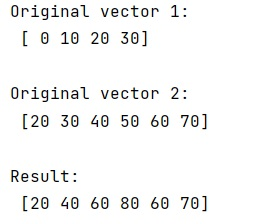# NumPy: Adding two vectors with different sizes

Given two vectors with different sizes, we have to add them using Python NumPy.
Submitted by Pranit Sharma, on March 06, 2023

## Add two vectors with different sizes

For example, if we have two vectors A and B as [0, 10, 20, 30] and [20, 30, 40, 50, 60, 70] and we need to add these vectors of different lengths.

To add two vectors with different sizes, we need to play with the length of both vectors and make some copies of the vectors.
We need to check if the length of A is less than B then we need to make a copy of B and then add the elements of A to it.

Also, if the length of B is less than A, we need to make a copy of A and add the elements of B to it.

Let us understand with the help of an example,

## Python code to add two vectors with different sizes

```# Import numpy
import numpy as np

# Creating vectors
v1 = np.array([0, 10, 20, 30])
v2 = np.array([20, 30, 40, 50, 60, 70])

# Display Original vectors
print("Original vector 1:\n",v1,"\n")
print("Original vector 2:\n",v2,"\n")

if len(v1) < len(v2):
res = v2.copy()
res[:len(v1)] += v1
else:
res = v1.copy()
res[:len(v2)] += v2

# Display result
print("Result:\n",res)
```

Output:Languages: » C » C++ » C++ STL » Java » Data Structure » C#.Net » Android » Kotlin » SQL
Web Technologies: » PHP » Python » JavaScript » CSS » Ajax » Node.js » Web programming/HTML
Solved programs: » C » C++ » DS » Java » C#
Aptitude que. & ans.: » C » C++ » Java » DBMS
Interview que. & ans.: » C » Embedded C » Java » SEO » HR
CS Subjects: » CS Basics » O.S. » Networks » DBMS » Embedded Systems » Cloud Computing
» Machine learning » CS Organizations » Linux » DOS
More: » Articles » Puzzles » News/Updates